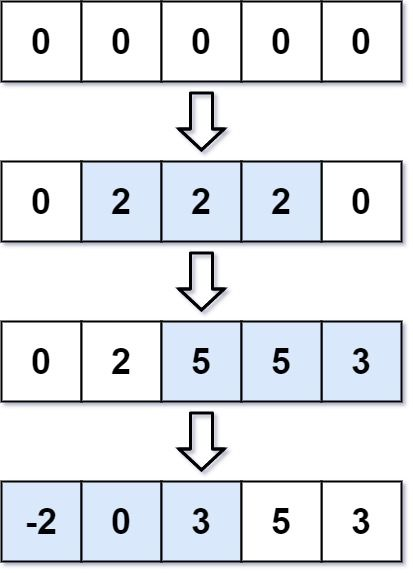# Question

Formatted question description: https://leetcode.ca/all/370.html

You are given an integer length and an array updates where updates[i] = [startIdxi, endIdxi, inci].

You have an array arr of length length with all zeros, and you have some operation to apply on arr. In the ith operation, you should increment all the elements arr[startIdxi], arr[startIdxi + 1], ..., arr[endIdxi] by inci.

Return arr after applying all the updates.

Example 1:Input: length = 5, updates = [[1,3,2],[2,4,3],[0,2,-2]]
Output: [-2,0,3,5,3]


Example 2:

Input: length = 10, updates = [[2,4,6],[5,6,8],[1,9,-4]]
Output: [0,-4,2,2,2,4,4,-4,-4,-4]


Constraints:

• 1 <= length <= 105
• 0 <= updates.length <= 104
• 0 <= startIdxi <= endIdxi < length
• -1000 <= inci <= 1000

# Algorithm

Suppose our array range is [0, n), the interval to be updated is [start, end], and the updated value is inc.

Then add inc to each number in the interval [start, end], which is equivalent to

• Add inc to all numbers in the interval (start, n)
• Then subtract inc from all the numbers in the interval (end+1, n)

After understanding that this conversion can be done, we still can’t update all the values in the interval every time, so we need to change the marking method.

• The method is to add inc to the start position at the beginning of the coordinate, and add -inc to the position where end adds 1.

For example, we need to add 2 to the numbers in the new interval [1, 3], then we add 2 at the position of 1, and subtract 2 at the position of 4, so the array becomes [0, 2, 0, 0, -2].

If there is only one operation, how to get the final result? The answer is to create a cumulative sum array, which becomes [0, 2, 2, 2, 0]

We found that it is exactly equivalent to directly adding 2 to the numbers in the interval [1, 3]. Further analysis, the operation of establishing the accumulation and array actually means that the current number has an effect on the numbers in all subsequent positions.

• Then we add 2 to the start position, which means that every number in the range of [start, n) is added 2
• In fact, only the numbers in the [start, end] interval need to be added by 2
• In order to eliminate this effect, we need to subtract 2 from the numbers in the [end+1, n) interval, so we subtract 2 from the end+1 position

Then when the accumulation and array is created, it is equivalent to subtracting 2 from all the numbers behind. It should be noted that end may be equal to n-1, then end+1 may be out of range, so we initialize the length of the array to n+1 to avoid the out of range.

Then according to the example in the title, we can get an array, nums = {-2, 2, 3, 2, -2, -3}, and then add it up and it is the result we require result = {-2, 0 , 3, 5, 3}

# Code

• import java.util.Arrays;

public static void main(String[] args) {
Solution s = out.new Solution();

System.out.println(Arrays.toString(s.getModifiedArray(5, new int[][]{ {1,3,2}, {2,4,3}, {0,2,-2} })));
}

class Solution {
public int[] getModifiedArray(int length, int[][] updates) {

int[] ans = new int[length];

for (int[] update : updates) {
int start = update;
int end = update;
int inc = update;

ans[start] += inc;
if (end + 1 < length) {
ans[end + 1] += -inc;
}

System.out.println(Arrays.toString(ans));
//                [0, 0, 0, 0, 0]
//                [0, 2, 0, 0, -2]
//                [0, 2, 3, 0, -2]
//                [-2, 2, 3, 2, -2]
}

for (int i = 1; i < length; i++) {
ans[i] += ans[i - 1]; // @note
}
//                [-2, 0, 3, 5, 3]

return ans;
}
}
}

############

class Solution {
public int[] getModifiedArray(int length, int[][] updates) {
int[] d = new int[length];
for (var e : updates) {
int l = e, r = e, c = e;
d[l] += c;
if (r + 1 < length) {
d[r + 1] -= c;
}
}
for (int i = 1; i < length; ++i) {
d[i] += d[i - 1];
}
return d;
}
}

• // OJ: https://leetcode.com/problems/range-addition/
// Time: O(N + U)
// Space: O(1)
class Solution {
public:
vector<int> getModifiedArray(int length, vector<vector<int>>& A) {
vector<int> ans(length);
for (auto &v : A) {
int start = v, end = v, diff = v;
ans[start] += diff;
if (end + 1 < length) ans[end + 1] -= diff;
}
int sum = 0;
for (auto &n : ans) n = sum += n;
return ans;
}
};

• class Solution:
def getModifiedArray(self, length: int, updates: List[List[int]]) -> List[int]:
d =  * length
for l, r, c in updates:
d[l] += c
if r + 1 < length:
d[r + 1] -= c
return list(accumulate(d))

############

class Solution(object):
"""
:type length: int
:rtype: List[int]
"""
ans =  * length
start, end, delta = update
ans[start] += delta
if end + 1 < length:
ans[end + 1] -= delta

delta = 0
for i in range(0, length):
delta += ans[i]
ans[i] = delta
return ans


• func getModifiedArray(length int, updates [][]int) []int {
d := make([]int, length)
for _, e := range updates {
l, r, c := e, e, e
d[l] += c
if r+1 < length {
d[r+1] -= c
}
}
for i := 1; i < length; i++ {
d[i] += d[i-1]
}
return d
}

• /**
* @param {number} length
* @return {number[]}
*/
var getModifiedArray = function (length, updates) {
const d = new Array(length).fill(0);
for (const [l, r, c] of updates) {
d[l] += c;
if (r + 1 < length) {
d[r + 1] -= c;
}
}
for (let i = 1; i < length; ++i) {
d[i] += d[i - 1];
}
return d;
};